Order of reaction

Order of reaction:- Sum of powers to which the concentration terms are raised in rate law expression

Order of reaction = ∝ + β,

Examples

If 0 → Zero order reaction

1 → Ist order reaction

2 → Second Order Reaction

1. Zero order reaction:- Rate is independent of concentration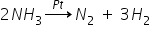Rate = K [NH3]°                                O.R = 0

Rate = K

2. First order reaction:- Rate depends only upon 1 concentration term.

2 N2O5 → 4 NO + 6 O2

Rate = K [N2O5]1                              O.R = 1

3. Second order reaction:- Rate depends upon 2 concentration terms.

2 NO2 + F2 → 2 NO2F

Rate = K [NO2]1 [F2]1                      O.R = 1 + 1 = 2

4. Third order reaction:- Rate depends upon 3 concentration terms.

2 NO + O2 → 2 NO2

Rate = K [NO2]2 [O2]1                     O.R = 2 + 1 = 3

5. Fractional order reaction:- Rate depends upon fractions of concentration terms.

CH3CHO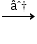CH4 + CO

Rate = K [CH3CHO]1.5                     O.R = 1.5

Units of rate constant [K] for 0, 1, 2, 3 order reactions

Rate = K ([Conc] / Time) = Mol L-1 Sec-1

For zero order:- Rate = K [A] °

Rate = K = mol lit-1 sec-1

For Ist order:- Rate = K [A]1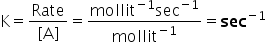For IInd order:- Rate = K [A]2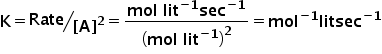For IIIrd order reaction:- Rate = K [A]3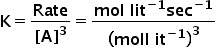= mol-2 lit2 sec-1

Question:- What is the order of reaction for

Rate = k [NO-2] [I-] [H+]2

Answer:- If concentration of H+ is doubled → 4 times

If concentration of NO-2 is Halved → ½ time

If concentration of NO-2, I- and H+ are tripled → 81 times

3 [NO-2]1 3 [I-]1 3 [H+]2 = 31 x 31 x 32 = 81

Question:- If rate of reaction becomes 27 times on increasing the concentration of reactant 3 times, what is the order of reaction.

Answer:- order of reaction is 3                                     flight → 3 [A]3O.R

33 = 3 x 3 x 3 = 27 times

Question:- What is the effect on rate of reaction, if volume of container is halved rate = K [A]2

Answer:- Rate of reaction is 4 times because concentration ∝ (1/volume)

In Case of gas, units are replaced by atm of place of mol lit-1. e.g.mol lit-1 sec-1 = atm sec-1

Question:- Calculate the units of K for rate = k [CH3CHO]3/2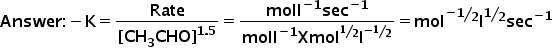Related Keywords